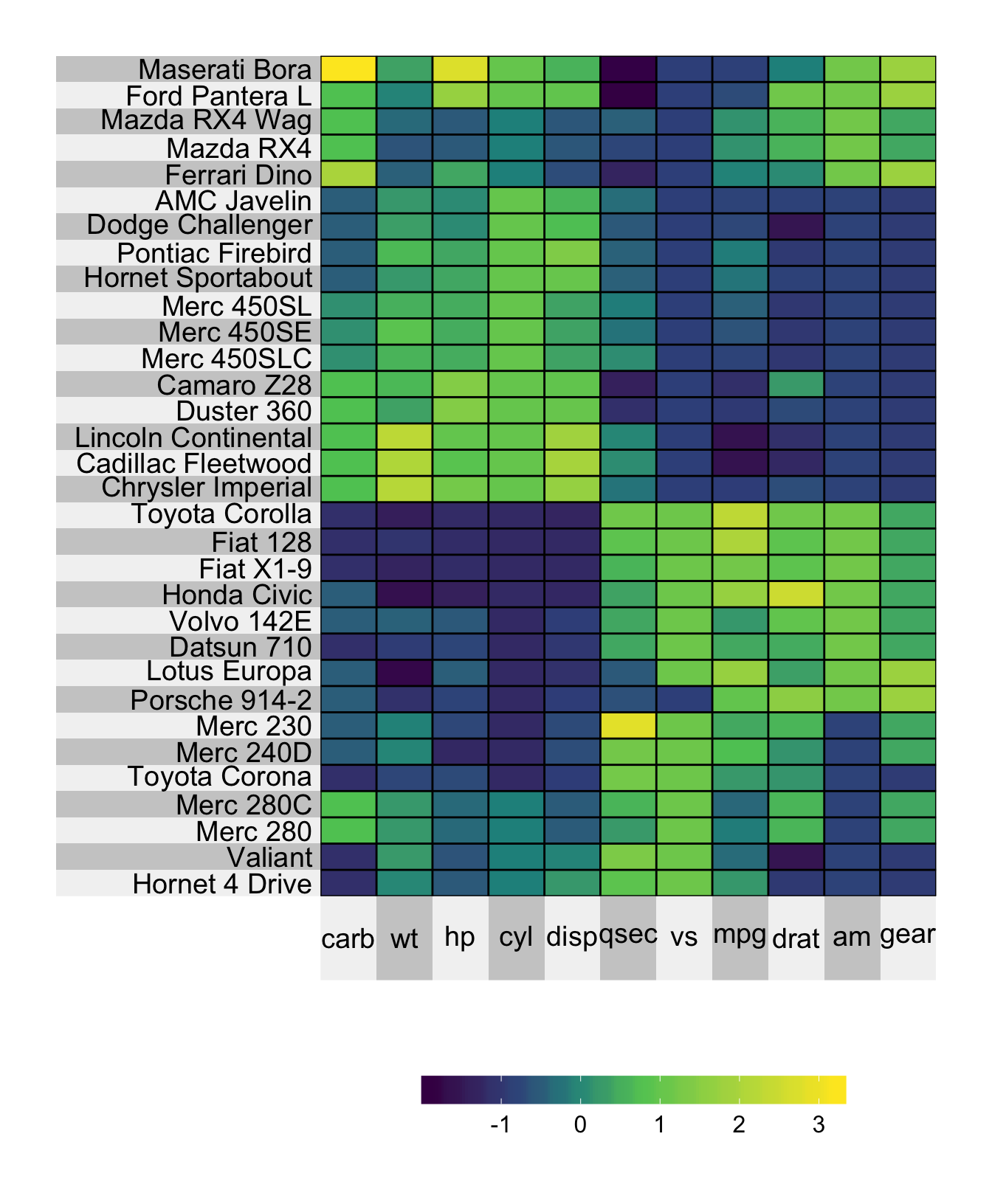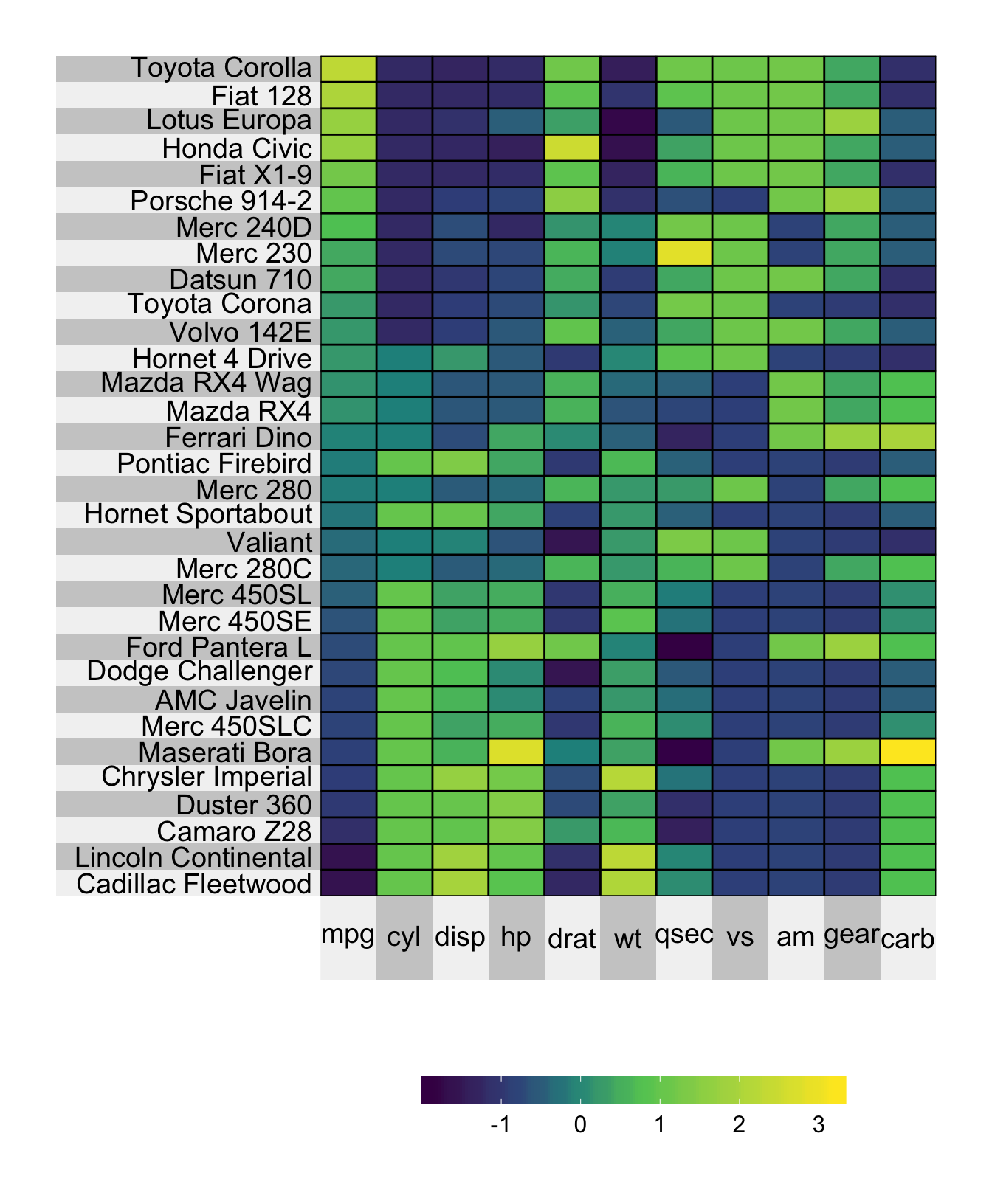# Chapter 3 Ordering rows and columns

By default, the rows and columns are ordered as in the original input.

## 3.1 Using hierarchical clustering to order the rows/columns

You can set the ordering of the rows/columns based on a “pretty” hierarchical clustering by specifying pretty.order.rows = TRUE and pretty.order.cols = TRUE. Errors may arise when the matrix has missing values.

# generate the plot:
superheat(mtcars,
# retain original order of rows/cols
pretty.order.rows = TRUE,
pretty.order.cols = TRUE,
# scale the matrix columns
scale = TRUE)## 3.2 Specifying the ordering of the columns or rows

If, instead, you would like to specify a custom ordering of the rows and columns, you can simply provide the order vector to the order.rows and order.cols arguments. In the example below, we order the rows by the mpg variable from the original matrix. Note that we could order by any vector i.e. we are not restricted to ordering by a column or row in our matrix.

# generate the plot:
superheat(mtcars,
# order the rows by miles per gallon
order.rows = order(mtcars\$mpg),
# scale the matrix columns
scale = TRUE)